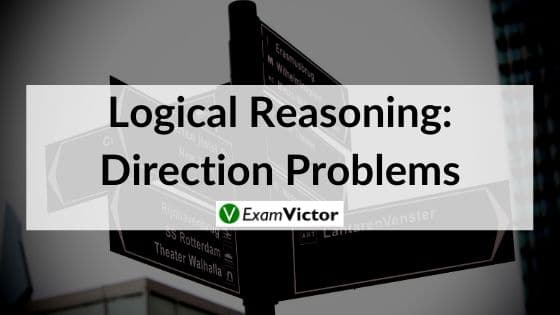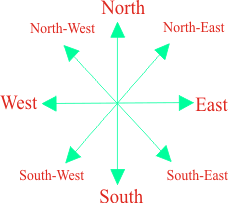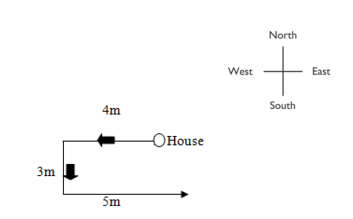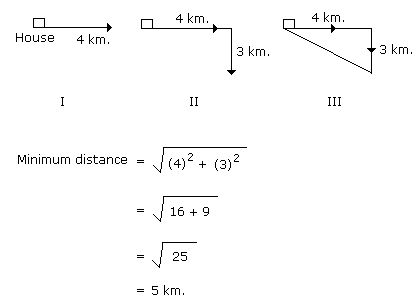# Logical Reasoning: Direction ProblemsLast Updated on Nov 4, 2020

Direction Problems under Logical Reasoning is one of the important topics. So, the concept should be clear to the students in depth. So, here we have explained this topic in a detailed and easy way. The direction problems are of two types, either they ask for distance or direction. You might be asked to calculate the distance or the direction. The foremost thing that you are supposed to do is draw basic direction map.#### Important points to remember

• When a question says moved towards the left or right side, we assume that the movement is at an angle of 90 degrees
• Keep in mind that when a person moves to his left side, he will move towards anti-clockwise direction and when a person moves to his right side, he will move towards the clockwise direction
• When a question says if someone moves towards the left or right side, we assume that the movement is at an angle of 90 degrees

Example-1: Aarthi walked 4 km west of her house and then turned south covering 3 km. Finally, She moved 5 km towards east. In which direction she is now with respect to the starting point?

Solution: From the above diagram, we can find that she is South East to her starting point.Example-2: Sandhya starting from her house, goes 4 km in the East, then she turns to her right and goes 3 km. What is the shortest distance to reach her house?

Solution

As we know that the shortest distance between these points will lie along the hypotenuse of the right-angled triangle formed by these points.After applying the Pythagoras theorem, the distance between point A and C is 5 km

### Summary

In this post, we have given some points to remember while solving Direction Problems under Logical Reasoning.  We have illustrated with diagrammatic examples to clear the concept. Click on the link given, if you would like to know about ‘Logical Reasoning: Statements & Assumptions’
Let us know your suggestions in the comment section below.